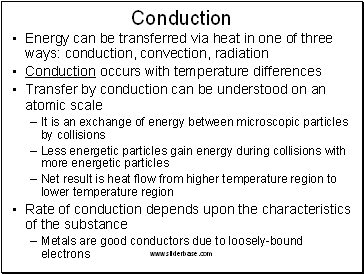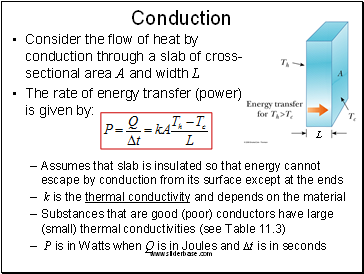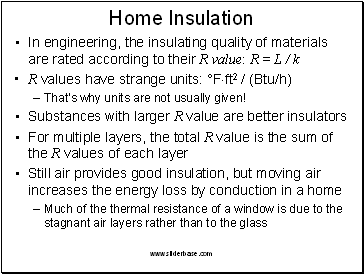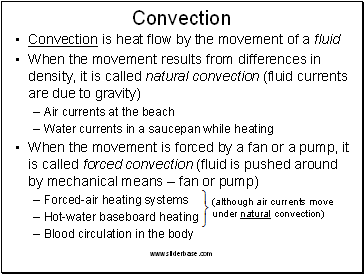# Thermal EnergyPage 4

#### WATCH ALL SLIDES

Reason why you feel cool after stepping out from a swimming pool

Slide 15Example Problem #11.31

Solution (details given in class):

16°C

A 40-g block of ice is cooled to –78°C and is then added to 560 g of water in an 80-g copper calorimeter at a temperature of 25°C. Determine the final temperature of the system consisting of the ice, water, and calorimeter. (If not all the ice melts, determine how much ice is left.) Remember that the ice must first warm to 0°C, melt, and then continue warming as water. The specific heat of ice is 0.500 cal/g°C = 2090 J/kg°C.

Slide 16## Conduction

Energy can be transferred via heat in one of three ways: conduction, convection, radiation

Conduction occurs with temperature differences

Transfer by conduction can be understood on an atomic scale

It is an exchange of energy between microscopic particles by collisions

Less energetic particles gain energy during collisions with more energetic particles

Net result is heat flow from higher temperature region to lower temperature region

Rate of conduction depends upon the characteristics of the substance

Metals are good conductors due to loosely-bound electrons

Slide 17Conduction

Consider the flow of heat by conduction through a slab of cross- sectional area A and width L

The rate of energy transfer (power) is given by:

Assumes that slab is insulated so that energy cannot escape by conduction from its surface except at the ends

k is the thermal conductivity and depends on the material

Substances that are good (poor) conductors have large (small) thermal conductivities (see Table 11.3)

P is in Watts when Q is in Joules and Dt is in seconds

L

Slide 18## Home Insulation

In engineering, the insulating quality of materials are rated according to their R value: R = L / k

R values have strange units: °Fft2 / (Btu/h)

That’s why units are not usually given!

Substances with larger R value are better insulators

For multiple layers, the total R value is the sum of the R values of each layer

Still air provides good insulation, but moving air increases the energy loss by conduction in a home

Much of the thermal resistance of a window is due to the stagnant air layers rather than to the glass

Slide 19## Convection

Convection is heat flow by the movement of a fluid

When the movement results from differences in density, it is called natural convection (fluid currents are due to gravity)

Go to page:
1  2  3  4  5  6